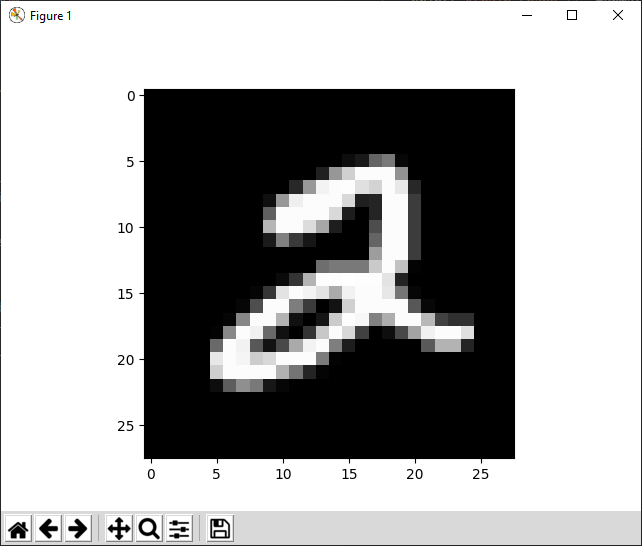# TensorFlow 筆記(2): MNIST dataset

Source: Deep Learning on Medium# MNIST dataset

MNIST是手寫數字的dataset, 裡面的每張圖片都是一個手寫數字圖片, 並且標示對應的數字。圖片的大小是28×28, 顏色格式是灰階

`import numpy as npimport tensorflow as tfimport matplotlib.pyplot as plt`

`(xtrain, ytrain), (xtest, ytest) = tf.keras.datasets.mnist.load_data()`

training data又分成xtrain, ytrain。xtrain是影像資料，ytrain是該圖片標示出對應的數字。xtest及ytest也是同樣地代表圖片以及對應的數字。

`print('xtrain.shape={}'.format(xtrain.shape))print('ytrain.shape={}'.format(ytrain.shape))print('xtest.shape={}'.format(xtest.shape))print('ytest.shape={}'.format(ytest.shape))'''xtrain.shape=(60000, 28, 28)ytrain.shape=(60000,)xtest.shape=(10000, 28, 28)ytest.shape=(10000,)'''`

`def plot_img(ndarr): img = ndarr.copy() img.shape = (28,28) plt.imshow(img, cmap='gray') plt.show()`

`plot_img(xtrain[5,:])`